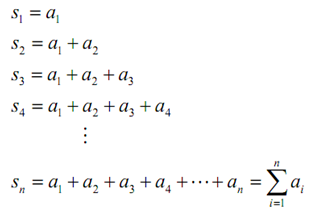## Basics of series - sequences and series, Mathematics

Assignment Help:

Series - The Basics

That topic is infinite series.  So just define what is an infinite series?  Well, let's start with a sequence {an}n=1 (note the n=1 is for convenience, it can be anything) and describe the following,The sn are called partial sums and notice that they will form a sequence, {sn}n=1.  Also recall that the ∑ is used to present this summation and termed as a variety of names. The most general names are:

a. Series notation,

b. Summation notation, and

c. Sigma notation.

#### LCM, What is the LCM of 4, 6, 18

What is the LCM of 4, 6, 18

#### Diffrential integral , All the integrals below are understood in the sense ...

All the integrals below are understood in the sense of the Lebesgue. (1) Prove the following equality which we used in class without proof. As-sume that f integrable over [3; 3]

#### Concept, uses of maths concept

uses of maths concept

Hi,Is this free?

#### Probability, what is a sample space diagram

what is a sample space diagram

#### Find the probability, Q. Suppose Jessica has 10 pairs of shorts and 5 pair...

Q. Suppose Jessica has 10 pairs of shorts and 5 pairs of jeans in her drawer. How many ways could she pick out something to wear for the day? What is the probability that she pick

#### Simulation and Modeling, I have some sample simulation and modeling practic...

I have some sample simulation and modeling practice questions using isee Stella software.

9:59-10:45

#### Evaluate the inverse function , Question: a. What is the inverse of f (...

Question: a. What is the inverse of f (x)? b. Graph the inverse function from part (a). c. Rewrite the inverse function from part (a) in exponential form. d. Evaluate

#### Evaluate the measure of the larger angle, Two angles are complementary. The...

Two angles are complementary. The calculate of one angle is four times the measure of the other. Evaluate the measure of the larger angle. a. 36° b. 72° c. 144° d. 18°